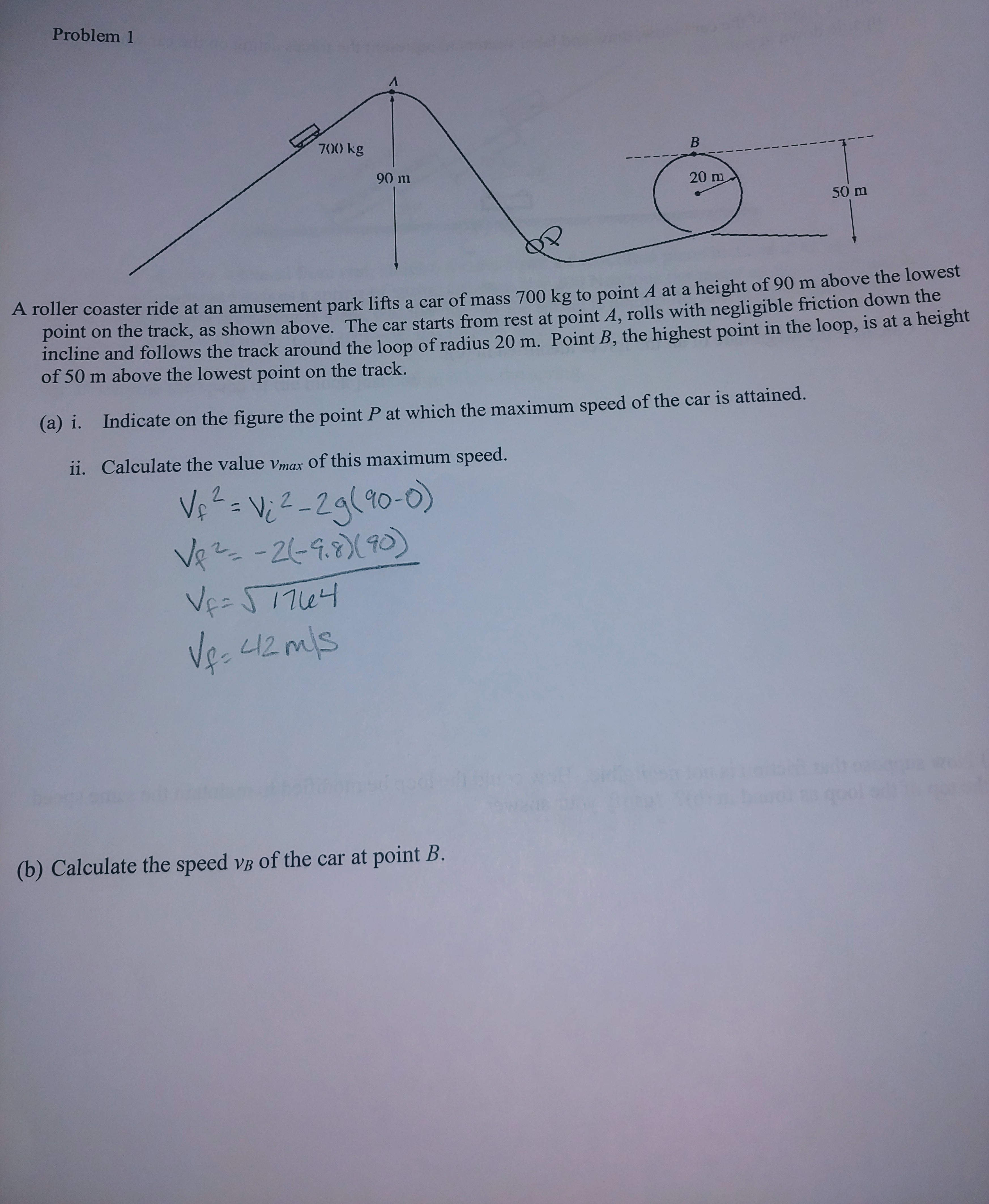# Problem 1700) kgВ90 m20 m50 mA roller coaster ride at an amusement park lifts a car of mass 700 kg to point A at a height of 90 m above the lowestpoint on the track, as shown above. The car starts from rest at point A, rolls with negligible friction down theincline and follows the track around the loop of radius 20 m. Point B, the highest point in the loop, is at a heightof 50 m above the lowest point on the track.Indicate on the figure the point P at which the maximum speed of the car is attained.(a) i.ii. Calculate the value vmax of this maximum speed.V 2-2g(90-0)-26-9.(0)Vee S17uVe-L1(b) Calculate the speed vB of the car at point B.

Question
261 views

I'm having some issues understanding my physics homework. It doesnt look like what we were doing in class and I dont think I'm using the right formulas. I need some help with a & b, attached.help_outlineImage TranscriptioncloseProblem 1 700) kg В 90 m 20 m 50 m A roller coaster ride at an amusement park lifts a car of mass 700 kg to point A at a height of 90 m above the lowest point on the track, as shown above. The car starts from rest at point A, rolls with negligible friction down the incline and follows the track around the loop of radius 20 m. Point B, the highest point in the loop, is at a height of 50 m above the lowest point on the track. Indicate on the figure the point P at which the maximum speed of the car is attained. (a) i. ii. Calculate the value vmax of this maximum speed. V 2-2g(90-0) -26-9.(0) Vee S17u Ve- L1 (b) Calculate the speed vB of the car at point B. fullscreen
check_circle

Step 1

(a)

(i) Point P, where the speed is maximum is located at the lowest point on the roller coaster. At the lowest point potential energy is lowest and hence kinetic energy is maximum.

Step 2

(ii) Write the expression for conservation of energy at point A and P

Step 3

Substituting th...

### Want to see the full answer?

See Solution

#### Want to see this answer and more?

Solutions are written by subject experts who are available 24/7. Questions are typically answered within 1 hour.*

See Solution
*Response times may vary by subject and question.
Tagged in

### Science